# ChIPS - displaying histogram dataChIPS Threads (CIAO 4.11 ChIPS v1)

## Overview

#### Synopsis:

This thread demonstrates some of the capabilities for displaying histogram data in ChIPS. This includes support for binned data that is given as either (xmid,y) or (xlo,xhi,y), errors on the Y value (symmetric or asymmetric), marking the bins with symbols, and coloring the histogram interior.

We also include other features in ChIPS, such as the support for multiple plots - including plots within plots - and for multiple axes within plots to allow different data to be shown on the same plot.

Last Update: 15 Dec 2015 - Updated for CIAO 4.8.

## Introduction

The Starting ChIPS thread describes how to start ChIPS. Please see the ChIPS GUI section of that thread for information on the ChIPS GUI.

The data used in this thread is available in the chips_data.tar.gz file.

```unix% ls -1 chips/histogram/
lc.fits
```

The plots used in this thread are based on the light curve data used in the CIAO filtering lightcurves thread, renamed to lc.fits. The contents of the file are:

```unix% dmlist lc.fits cols

--------------------------------------------------------------------------------
Columns for Table Block LIGHTCURVE
--------------------------------------------------------------------------------

ColNo  Name                 Unit        Type             Range
1   TIME_BIN             channel      Int4           1:153                S/C TT corresponding to mid-exposure
2   TIME_MIN             s            Real8          77377470.9499280006: 77408016.4635529965 Minimum Value in Bin
3   TIME                 s            Real8          77377470.9499280006: 77408016.4635529965 S/C TT corresponding to mid-exposure
4   TIME_MAX             s            Real8          77377470.9499280006: 77408016.4635529965 Maximum Value in Bin
5   COUNTS               count        Int4           -                    Counts
6   STAT_ERR             count        Real8          0:+Inf               Statistical error
7   AREA                 pixel**2     Real8          -Inf:+Inf            Area of extraction
8   EXPOSURE             s            Real8          -Inf:+Inf            Time per interval
9   COUNT_RATE           count/s      Real8          0:+Inf               Rate
10   COUNT_RATE_ERR       count/s      Real8          0:+Inf               Rate Error

--------------------------------------------------------------------------------
World Coord Transforms for Columns in Table Block LIGHTCURVE
--------------------------------------------------------------------------------

ColNo    Name
2:    DT_MIN               = +0 [s] +1.0 * (TIME_MIN  -77377570.949928)
3:    DT                   = +0 [s] +1.0 * (TIME  -77377570.949928)
4:    DT_MAX               = +0 [s] +1.0 * (TIME_MAX  -77377570.949928)

```

## Drawing a histogram of xmid,y data values

The histogram support in ChIPS allows you to draw a histogram of previously-binned data - given as either xmid,y or xlo,xhi,y - but does not perform the binning for you. The creation of the histogram can be performed by CIAO tools like dmextract and dmcopy, or by routines in the Python language (in particular those from NumPy).

In this section we show how you can use make_figure and add_histogram routines to draw histograms of the DT and COUNT_RATE columns from lc.fits.

```chips> make_figure("lc.fits[cols dt,count_rate]", "histogram")
chips> print(info())
Window [win1]
Frame [frm1]
Plot [plot1]   (0.15,0.15)  .. (0.90,0.90)
Border bottom [bx1]  top [bx2]  left [by1]  right [by2]
X Axis [ax1]
Y Axis [ay1]
Histogram [hist1]

chips> print_window("basic")
chips> print_window("basic.pdf")
chips> print_window("basic.png")
```

### Figure 1: A basic histogram created by make_figure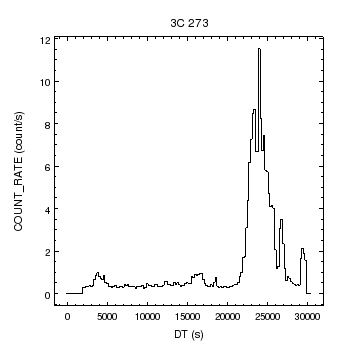### Figure 1: A basic histogram created by make_figure

The make_figure command has drawn a histogram of the DT and COUNT_RATE columns from the file lc.fits.

Unlike the case of creating curves (for example, when creating the latitude versus time figure), the figuretype argument to make_figure must be supplied when creating histograms.

We now show how the same data is displayed as a curve, to highlight the differences in appearance and behavior of the two objects in ChIPS. To make it easier to compare the two, we add a second plot, arranged vertically below the first one, using the split command, and add the curve to it. To remove the overlapping labels we remove the title of the second - i.e. bottom plot - and the X-axis label of the first plot by setting them both to "". The result is shown in Figure 2.

```chips> split(2)
chips> make_figure("lc.fits[cols dt,count_rate]", ["symbol.style", "none"])
chips> set_plot_title("")
chips> set_plot_xlabel("plot1", "")
chips> print(info())
Window [win1]
Frame [frm1]
Plot [plot1]   (0.15,0.52)  .. (0.90,0.90)
Border bottom [bx1]  top [bx2]  left [by1]  right [by2]
X Axis [ax1]
Y Axis [ay1]
Histogram [hist1]
Plot [plot2]   (0.15,0.15)  .. (0.90,0.52)
Border bottom [bx1]  top [bx2]  left [by1]  right [by2]
Curve [crv1]
X Axis [ax1]
Y Axis [ay1]

chips> save_state("compare1.state")
```

### Figure 2: Comparing histograms and curves### Figure 2: Comparing histograms and curves

Data in x,y format can be drawn as a histogram (top plot) or a curve (bottom plot).

A careful inspection of the X-axes in Figure 2 shows that they do not quite line up; the histogram plot covers a slightly-larger range, as also shown by the return value of the get_plot_xrange calls.

```chips> get_plot_xrange("plot1")
[-1630.0, 32030.0]
chips> get_plot_xrange("plot2")
[-1520.0, 31920.0]
```

The reason for this difference is because the histogram is drawn from the start to end of each bin, whereas the curve uses the mid-point of the bin. This is graphically shown in Figure 3, which was created using the following commands:

```chips> clear()
chips> add_histogram("lc.fits[cols dt,count_rate]")
chips> add_curve("lc.fits[cols dt,count_rate]")
chips> set_curve(["line.color", "red", "line.style", "dot"])
chips> set_curve(["symbol.color", "green", "symbol.style", "circle"])
chips> limits(X_AXIS, 27000, 31000)
chips> limits(Y_AXIS, -0.2, 2.5)
chips> print(info())
Window [win1]
Frame [frm1]
Plot [plot1]   (0.15,0.15)  .. (0.90,0.90)
Border bottom [bx1]  top [bx2]  left [by1]  right [by2]
X Axis [ax1]
Y Axis [ay1]
Histogram [hist1]
Curve [crv1]

```

### Figure 3: How are curves and histograms displayed?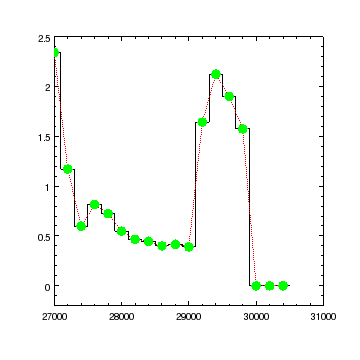### Figure 3: How are curves and histograms displayed?

The red dotted line and green circles indicate the curve, whereas the solid line (white on screen, black in the hardcopy output) is the histogram. The curve ends at the last point (x = 30400) whereas the histogram extends past this to the edge of the bin (x = 30500).

## Controlling the appearance of histograms

As with curves, the appearance of histograms can be controlled by a set of attributes. The get_histogram and set_histogram routines are used to report and change these attributes. In the example below we change the histogram to be drawn in blue, and force each bin edge to be drawn down to y=0 (the default setting is to only do this for the start and end bins). The resulting figure is shown in Figure 4.

```chips> clear()
chips> add_histogram("lc.fits[cols dt,count_rate]")
chips> print(get_histogram())
depth = 100
dropline = False
err.caplength = 10
err.color = default
err.down = False
err.style = line
err.thickness = 1.0
err.up = False
fill.color = default
fill.opacity = 1.0
fill.style = 0
id = None
line.color = default
line.style = 1
line.thickness = 1.0
stem = None
symbol.angle = 0.0
symbol.color = default
symbol.fill = False
symbol.size = 5
symbol.style = 0

chips> set_histogram(["line.color", "blue", "dropline", True])
```

The ChIPS GUI makes it easy to explore and modify your ChIPS visualizations.

### Figure 4: Changing the line used to draw the histogram### Figure 4: Changing the line used to draw the histogram

The dropline attribute, when set to True, causes all bin edges of the histogram to be drawn down to y=0. When set to False, only the start of the first bin and end of the last bin are forced to be drawn like this.

The default attribute settings for histograms can be found by saying:

```chips> print(get_preference("histogram"))
histogram.stem         : hist
histogram.depth        : default
histogram.line.color   : default
histogram.line.thickness: 1
histogram.line.style   : solid
histogram.symbol.color : default
histogram.symbol.style : none
histogram.symbol.size  : 5
histogram.symbol.angle : 0
histogram.symbol.fill  : false
histogram.err.color    : default
histogram.err.thickness: 1
histogram.err.style    : line
histogram.err.up       : on
histogram.err.down     : on
histogram.err.caplength: 10
histogram.dropline     : off
histogram.fill.color   : default
histogram.fill.opacity : 1
histogram.fill.style   : nofill
```

We now remove the last change, using undo, and then set the fill.style attribute to "solid" so that the histogram is drawn with a filled interior, which we also set to be red. The result is shown in Figure 5.

```chips> undo()
chips> set_histogram(["fill.color", "red", "fill.style", "solid"])
chips> log_scale(Y_AXIS)
```

### Figure 5: A filled histogram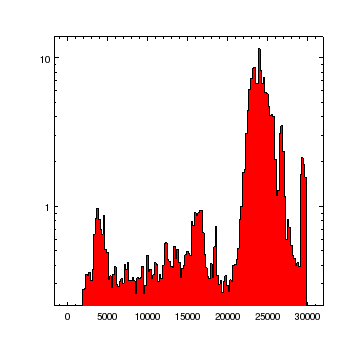### Figure 5: A filled histogram

The fill.* attributes of a histogram determine if, and how, the histogram is to be filled. In this case the interior is set to be filled with a solid red. Since the fill.opacity attribute is set to 1, the fill pattern is fully opaque, which is why the tick labels along the X axis are hidden by the histogram.

The y limits of the plot are based on the range of the positive y values, in this case 0.25 to 11.5 (see dmstat output below), since we have changed the plot to use a logarithmic scale for the Y axis.

```chips> !dmstat "lc.fits[count_rate > 0][cols count_rate]" cen- | egrep 'min|max'
min:        0.258270375           @:        34
max:        11.520884375          @:        111
chips> get_plot_yrange()
[0.21360136902802859, 13.930168806513759]
```

As the get_plot_yrange() output shows, the actual limits are slightly different to the data values due to the axis.pad setting of 0.05, which adds 5 per cent of the data range to the lowest and highest data values being displayed.

Filled and opaque histograms and regions display correctly in the PNG and JPEG formats created by print_window. For PS and PDF outputs the faint structures (lines) may still be visible on screen, but should not appear when the file is printed out.

There are two ways to stop the filled region from overlapping the X axis in Figure 5. The first option - shown in Figure 6 - is to reduce the fill.opacity setting from 1:

```chips> set_histogram(["fill.opacity", 0.4])
chips> save_state("attr-fill-opaque.state")
```

### Figure 6: Reducing the opacity of a filled histogram### Figure 6: Reducing the opacity of a filled histogram

By reducing the opacity of the histogram fill - which is controlled by the fill.opacity attribute - the tick labels on the X axis can be seen, although they are tinted red.

The opacity setting of histogram and region fills, and alpha setting of images, is supported in all output formats except for PS and EPS: compare the PDF and PS outputs.

The alternative, shown in Figure 7, is to move the histogram so that it is drawn before the axes. One way to do this is to change the depth attribute of the histogram so that it is less than all other elements of the plot. An example of how to do this is shown when creating Figure 9 of the "symbol support in curves" thread. The other way is to shuffle the histogram backwards so that it is drawn behind all the other elements at its depth (the call to undo is just to remove the opacity change used to create Figure 6):

```chips> undo()
chips> shuffle_histogram(chips_back)
chips> print(info_depth())
Window [win1]
Frame [frm1]
Depth 100
histogram [hist1 (plot1)]
label [title (plot1)]
axis [bx1 (plot1)]
axis [bx2 (plot1)]
axis [by1 (plot1)]
axis [by2 (plot1)]
axis [ax1 (plot1)]
axis [ay1 (plot1)]

chips> save_state("attr-fill-back.state")
```

### Figure 7: Moving the histogram behind other objects### Figure 7: Moving the histogram behind other objects

The histogram is drawn first among all the objects with the same depth and so no-longer obscures the tick labels of the X axis (compare to Figure 5).

We now change the fill pattern from "solid" to "updiagonal", with the result shown in Figure 8:

```chips> set_histogram(["fill.style", "updiagonal"])
```

### Figure 8: Changing the histogram to be filled with a non-solid pattern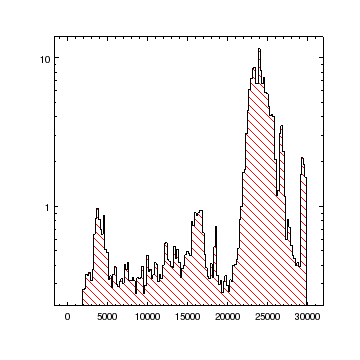### Figure 8: Changing the histogram to be filled with a non-solid pattern

The fill pattern has changed from being a solid color to a set of equally-spaced lines, going from lower left to upper right.

In CIAO 4.8 the PNG and JPEG outputs may swap the updiagonal and downdiagonal patterns (i.e. the PNG version of this plot uses the downdiagonal pattern).

## Adjusting the axis of a plot

So far we have used the DT column of the light curve since , as shown in Figure 9, using the TIME values for Chandra data can easily produce confusing plots, as the X-axis values are large. In this section we look at how we can improve the appearance of an axis in such a situation. First we need to create the initial histogram:

```chips> clear()
chips> add_histogram("lc.fits[cols time,count_rate]")
chips> set_plot_xlabel("Time (s)")
chips> set_plot_ylabel("Count Rate (count s^{-1})")
```

### Figure 9: Overlapping X-axis tick labels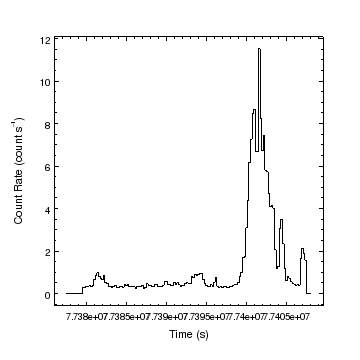### Figure 9: Overlapping X-axis tick labels

Since the TIME values are large (close to 7.74 x 107 s), the default settings for the X axis cause the tick labels to overlap.

The position and spacing of the major tick marks, and hence the tick labels, are controlled by the majortick.* attributes of an axis. The default value for the majortick.mode attribute is limits, as shown below, which means that the axis limits are based on the data values or set by the user, and the tick marks are automatically calculated to cover this range. Note that the format for the ticklabels is controlled by the tickformat attribute of the axis (here it is set to %g).

```chips> print(get_xaxis().majortick)
color = default
count = 6
interval = 10.0
length = 4
mode = limits
style = inside
thickness = 1.0
visible = True

chips> get_xaxis().tickformat
'%g'
```

We can change the tick spacing by several means; here we are going to change the majortick.interval attribute (which will automatically change the majortick.mode to interval) to use a spacing of 10 ks. This gives us three major tick marks on the X axis, as shown in Figure 10.

```chips> xr = get_plot_xrange()
chips> print(xr)
[77375940.949928, 77409600.949928]
chips> print(xr - xr)
33660.0
chips> set_xaxis(["majortick.interval", 1e4])
```

### Figure 10: Setting the spacing between major tick marks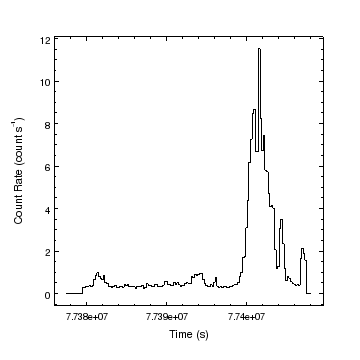### Figure 10: Setting the spacing between major tick marks

By changing the majortick.mode mode of the X axis from limits to interval, and setting the interval to a large fraction of the axis range, the tick labels no longer overlap.

We can change the format used to create the labels; here we remove the scientific notation and avoid displaying any decimal places by setting the format to "%.0f". There are a wide range of ticklabel formats supported by ChIPS.

```chips> set_xaxis(["tickformat", "%.0f"])
```

Setting the majortick.count attribute determines the number of major tick marks. The axis limits may be increased by this algorithm, as they are here (Figure 11). The number of minor tick marks has also been reduced to 1 below.

```chips> set_xaxis(["majortick.count", 5, "minortick.count", 1])
chips> get_plot_xrange()
[77370000.0, 77410000.0]
```

### Figure 11: Using the count major tick mode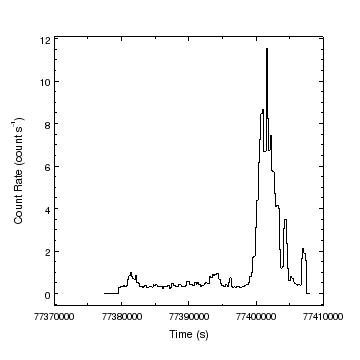### Figure 11: Using the count major tick mode

By changing to the count mode for the major tick mode, the X-axis limits have been automatically increased.

With the mode set to count, the X-axis may not change when the limits are changed, as shown below (after the limits call the display remains showing Figure 11).

```chips> limits(X_AXIS, 7.7376e7, 7.741e7)
chips> get_plot_xrange()
[77370000.0, 77410000.0]
```

Changing back to the limits mode means that the X-axis will match the settings you give, and so the plot changes to the values given above (see Figure 12).

```chips> set_xaxis(["majortick.mode", "limits"])
chips> get_plot_xrange()
[77376000.0, 77410000.0]
```

### Figure 12: Switching back to the limits mode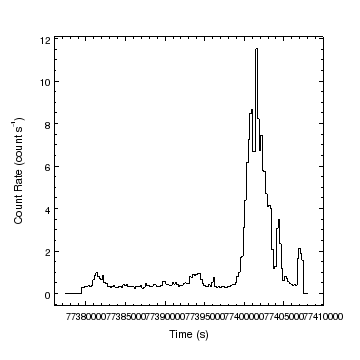### Figure 12: Switching back to the limits mode

When using the limits mode - which is the default value for the majortick.mode attribute - the axis will match the range given in the limits call.

## Histograms of data with low and high values for the bin edges

In this section we will show you how to display data which has low and high bin edges, rather than use the middle of the bin as we did earlier. For this we need to send data arrays to the add_histogram call, rather than a file name. So first we read in the data from the file lc.fits using the Crates module:

```chips> cr = read_file("lc.fits")
chips> print(get_col_names(cr, rawonly=False))
['TIME_BIN', 'TIME_MIN', 'TIME', 'TIME_MAX', 'COUNTS', 'STAT_ERR', 'AREA', 'EXPOSURE', 'COUNT_RATE', 'COUNT_RATE_ERR', 'DT_MIN', 'DT', 'DT_MAX']
chips> xlo = copy_colvals(cr, "dt_min")
chips> xhi = copy_colvals(cr, "dt_max")
chips> y = copy_colvals(cr, "count_rate")
chips> dy = copy_colvals(cr, "count_rate_err")
```

If given two arrays then add_histogram will assume the X array refers to the mid-point of each bin. However, if given three arrays it uses the first two arrays to define the start and edge of each bin. The resulting figure is shown in Figure 13:

```chips> clear()
chips> add_histogram(xlo, xhi, y)
```

### Figure 13: A histogram of xlo,xhi,y values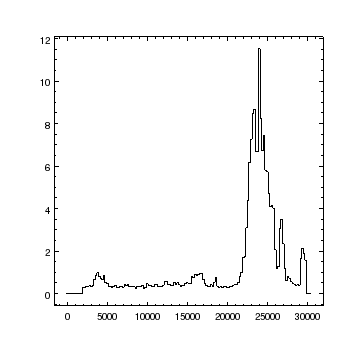### Figure 13: A histogram of xlo,xhi,y values

This figure shows the histogram created by plotting up the DT_MIN, DT_MAX columns - which determine the bin edges - against the COUNT_RATE column.

The result is the same as Figure 9 - except for the axis labels - since for this data set the time bins all have the same width and are contiguous. This can be seen by overplotting the histogram calculated using the DT values (see Figure 14).

```chips> xmid = copy_colvals(cr, "dt")
chips> add_histogram(xmid, y, ["line.color", "red"])
```

### Figure 14: Comparing histograms### Figure 14: Comparing histograms

The red histogram - which represents the DT,COUNT_RATE values - is the same as the one calculated from the DT_MIN,DT_MAX bin edges.

We could use undo to remove the second histogram, but we decide to delete it instead using delete_histogram.

```chips> delete_histogram()
chips> set_histogram(["symbol.style", "circle"])
```

The pick and get_pick routines can be used if you want to select a point or region in a plot. When called with no arguments, pick will report the location of the first click you make in the plot. During this time it may update the title of the ChIPS window with the current location of the mouse (this feature depends on the window manager being used). In the output below we selected a point near the peak of the light curve. The get_pick routine is used if you want the coordinates of the point, or points, returned in Python variables.

```chips> pick()
(23851.9, 11.6088)
```

Note that the mouse coordinates are displayed along with the window title whenever the ChIPS window is active and the mouse within the window (as long as your window manager displays the title bar of windows).

Here we decide to restrict the X axis of the plot, mainly to remove the zero values at the start and end of the time series. The result is Figure 15.

```chips> limits(X_AXIS, 12000, 27500)
```

The pick_limits command allows you to interactively chose the limits of a plot by selecting the corners of the new axis ranges with the mouse. So after entering

```chips> undo()
chips> pick_limits()
```

move the mouse into the ChIPS plot window, select one corner of the plot with the left mouse button, and - whilst holding down the button - drag to the other corner. A rectangle will be drawn during the mouse drag to indicate the new plot limits. Once the button has been released the axes limits will be changed. To quit the zoom without changing the limits press the Escape key.

It is also possible to directly interact with the ChIPS window, with support for panning, dragging, and zooming of the plot limits using the mouse (or, for some functionality, the keyboard).

### Figure 15: Adding symbols to histograms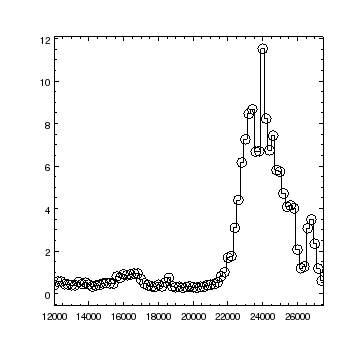### Figure 15: Adding symbols to histograms

Histograms can also be drawn using symbols.

The support for symbols is the same as for curves. Here we show how multiple values can be changed with a single call to set_histogram using the value returned by get_histogram. The result is Figure 16.

```chips> limits(X_AXIS, 12000, 27500)
chips> limits(Y_AXIS, AUTO, AUTO)
chips> log_scale(Y_AXIS)
chips> hi = get_histogram()
chips> hi.symbol.color = "red"
chips> hi.symbol.fill = True
chips> hi.symbol.size = 3
chips> set_histogram(hi)
chips> save_state("sym-circle-fill.state")
```

### Figure 16: Changing symbol properties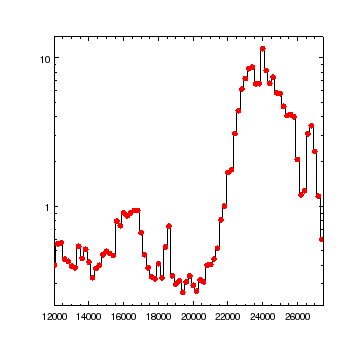### Figure 16: Changing symbol properties

The symbols are now drawn as red, filled circles.

## Error bars

Error bars on the Y axis values, either symmetric or asymmetric, can be included in histograms. Here we add the errors on the count-rate measurements, which are quite small for this data set, as Figure 17 shows.

```chips> clear()
chips> add_histogram(xlo, xhi, y, dy)
chips> limits(2400, 12500, 0, 1.5)
```

### Figure 17: Displaying error bars on a histogram### Figure 17: Displaying error bars on a histogram

As with curves, histograms can display error bars, although only for the Y axis values.

The line can be removed, by setting the line.style attribute to noline (Figure 18):

```chips> set_histogram(["line.style", "noline"])
```

### Figure 18: A histogram without lines### Figure 18: A histogram without lines

The line style can be set to noline (or none) to remove the line that connects the bins.

Symbols can be added (here an open square), which creates Figure 19:

```chips> set_histogram(["symbol.style", "square"])
```

### Figure 19: Symbols and error bars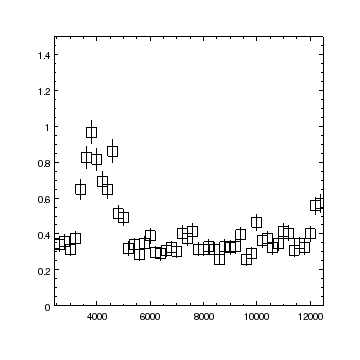### Figure 19: Symbols and error bars

An open square has been chosen to mark the mid-point of each bin.

Error bars can be added to histograms specified just using the mid-point of the bin, but you still have to supply two arguments for the low and high bin edges, but set the second one to None. First we create the data to plot; we chose those bins which have a positive count rate and convert the time values from seconds to kiloseconds. We use the Crates routine get_keyval to get the OBJECT keyword from the data set.

```chips> i = y > 0
chips> x2 = xmid[i] / 1e3
chips> y2 = y[i]
chips> dy2 = dy[i]
chips> obj = get_keyval(cr, "OBJECT")
```

Now we have the data we can plot it. The first argument to add_histogram is the bin center and the second argument is None, which means the histogram bins are taken to be centered at x2,y2.

```chips> clear()
chips> add_histogram(x2, None, y2, dy2, ["err.color", "red", "line.color", "blue"])
chips> log_scale(Y_AXIS)
chips> set_plot_xlabel(r"\Delta t (ks)")
chips> set_plot_ylabel("Rate (count s^{-1})")
chips> set_plot_title(obj)
chips> save_state("err-mid.state")
```

### Figure 20: Error bars for xmid,y histograms### Figure 20: Error bars for xmid,y histograms

Colors have been added to show that the individual elements of a histogram can be colored separately.

## Zooming in on a histogram

In this section we will add a second plot to Figure 20, showing a zoomed-in region of the start of the light curve (before the flare). To make the most use of the space we will manually add the second plot so that it appears within the existing plot. We do this by calling add_plot to create the new plot and give it the coordinates to use for the bottom-left and top-right of the plot. The coordinate system used here is "frame normalized", where 0,0 is the bottom-left of the frame and 1,1 is the top-right of the frame. Note that undo, move_plot, and reposition_plot are useful commands when trying to get the plot in a good position. The result of the add_plot call is shown in Figure 21.

```chips> add_plot(0.25, 0.5, 0.6, 0.85, ["id", "zoom"])
chips> print(get_plot())
bottommargin = 0.5
corner.style = miter
id = None
leftmargin = 0.25
rightmargin = 0.399999976158
stem = None
style = closed
title.angle = 0.0
title.color = default
title.depth = 100
title.font = helvetica
title.fontstyle = normal
title.halign = 0.5
title.size = 16
title.valign = 0.5
title.xpos = 0.5
title.ypos = 15.0
topmargin = 0.149999976158
```

Note that the rightmargin and topmargin values are set to values of 1-xpos and 1-ypos, where xpos and ypos are the second pair of coordinates used in the add_plot call above (with some small rounding error).

### Figure 21: Placing a plot within an existing plot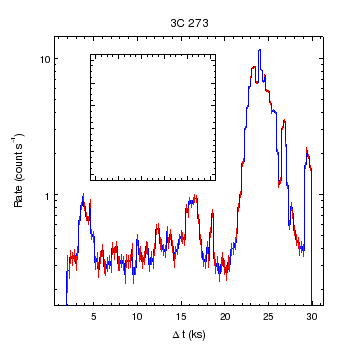### Figure 21: Placing a plot within an existing plot

The second plot - shown as just the borders - is placed in the top-left corner of the first plot.

Plots - and many other objects - can also be directly re-sized or moved using the ChIPS GUI.

We decide that we do not want borders around the plot, so we change the plot style from closed to open. After the set_plot call, the plot is no longer visible (i.e. the result looks like Figure 20), but the plot still exists, as is shown by the output of info:

```chips> set_plot(["style", "open"])
chips> print(info())
Window [win1]
Frame [frm1]
Plot [plot1]   (0.15,0.15)  .. (0.90,0.90)
Border bottom [bx1]  top [bx2]  left [by1]  right [by2]
X Axis [ax1]
Y Axis [ay1]
Histogram [hist1]
Plot [zoom]   (0.25,0.50)  .. (0.60,0.85)
Border bottom [bx1]  top [bx2]  left [by1]  right [by2]

chips> print(info_current())
Window [win1]
Frame [frm1]
Plot [zoom]
Coord Sys [Plot Normalized]

```

As the second plot is current, any new histogram will be added to it (and will automatically create axes, since the plot zoom does not contain any yet). We change the id from the default (which would be hist1) to make it clearer which objects are current in the output of info and info_current (and also to show that you can have different objects with the same id, in this case a plot and a histogram).

```chips> add_histogram(x2, y2, ["id", "zoom"])
chips> print(info())
Window [win1]
Frame [frm1]
Plot [plot1]   (0.15,0.15)  .. (0.90,0.90)
Border bottom [bx1]  top [bx2]  left [by1]  right [by2]
X Axis [ax1]
Y Axis [ay1]
Histogram [hist1]
Plot [zoom]   (0.25,0.50)  .. (0.60,0.85)
Border bottom [bx1]  top [bx2]  left [by1]  right [by2]
X Axis [ax1]
Y Axis [ay1]
Histogram [zoom]

chips> print(info_current())
Window [win1]
Frame [frm1]
Plot [zoom]
X Axis [ax1]
Y Axis [ay1]
Histogram [zoom]
Coord Sys [Data]
Coord Sys ID [ds6.6.8.27]

```

We zoom in on the region of interest, and make the histogram filled with the following three commands, to create Figure 22:

```chips> limits(1, 22, 0, 1.5)
chips> set_histogram(["fill.style", "solid"])
```

### Figure 22: Zooming in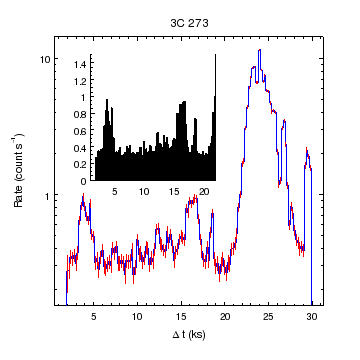### Figure 22: Zooming in

The second plot shows the start of the lightcurve; since the flare is not included in this time period the Y-axis has been left using a linear scale.

As the second histogram has been drawn filled, the X-axis tick marks are not visible in this plot. We could change the Y axis so that it starts at a negative value, such as -0.1, since the histogram fill extends down to y=0, but we decide to change the color of the axes from the default value to gray, and then shuffle the X axis so that it is drawn above all the other elements at its depth (so it will not be over-drawn by the histogram). Since an axis contains several color attributes - e.g. the axis itself, the major and minor tick marks, the label - we make use of the *.color attribute to change all the color settings at once. As no axis id was given, the changes are applied to both the X and Y axes, as these are both current (see the output of the info_current command above). The result is shown in Figure 23.

```chips> set_axis(["*.color", "gray"])
chips> shuffle_axis("ax1", chips_front)
chips> save_state("pip.state")
```

### Figure 23: Re-displaying the X axis### Figure 23: Re-displaying the X axis

Compare the axes in the second plot to those in Figure 22; the tick labels are now visible on the X axis and the axes are now drawn in gray (the color difference may be more visible in the PNG and postscript versions of the figure).

## Using two Y axes in a single plot

In this section we show how you can add an extra axis to a plot and use it to display a second set of data. This is different to the Customizing the axes section of the "symbol support in curves" thread, where a second axis was added for informational purposes.

Our aim is to plot up the count-rate histogram of lc.fits and overlay a curve showing the exposure time of each bin, which is stored in the EXPOSURE column of the file. We therefore get this column from the crate and filter it to remove non-zero times to create the array e2:

```chips> etime = copy_colvals(cr, "EXPOSURE")
chips> e2 = etime[i]
```

First we plot up the histogram, using as the X-axis values the offset in kiloseconds array (x2) created in the errors section above.

```chips> clear()
chips> add_histogram(x2, y2)
chips> set_plot_xlabel(r"\Delta t (ks)")
chips> set_plot_ylabel("Rate (count s^{-1})")
```

Now we add a second Y axis to the plot. We chose to position it at the right-hand edge of the plot, which means we can use the plot-normalized coordinate system (which is the default system for add_axis) and set the coordinate to 1 The minimum and maximum values for the new axis are set to 0 and 1 respectively; the values are not important as they will be changed when the exposure curve is added later. The result of these commands is Figure 24.

```chips> add_axis(Y_AXIS, 1, 0, 1)
chips> print(info())
Window [win1]
Frame [frm1]
Plot [plot1]   (0.15,0.15)  .. (0.90,0.90)
Border bottom [bx1]  top [bx2]  left [by1]  right [by2]
X Axis [ax1]
Y Axis [ay1]
Histogram [hist1]
Y Axis [ay2]

chips> print(info_current())
Window [win1]
Frame [frm1]
Plot [plot1]
X Axis [ax1]
Histogram [hist1]
Y Axis [ay2]
Coord Sys [Data]
Coord Sys ID [ds7.7.9.31]

```

### Figure 24: Adding a second Y axis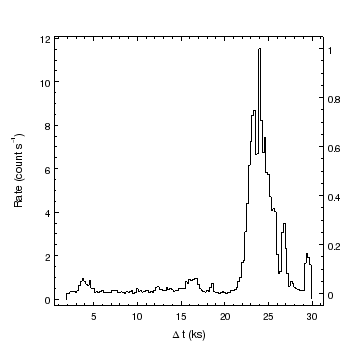### Figure 24: Adding a second Y axis

The new Y axis has been added to the right-hand edge of the plot. Since it has been added in plot-normalized coordinates then it will move with the plot - as shown below when we increase the rightmargin attribute of the plot to create Figure 26 - but will not move if we change the X-axis limits of the plot.

The axes used to plot a curve or histogram (i.e. the data coordinate system that is used) is determined by the current pair of axes. The output of info_current above show that the current axes are the X axis and the second Y axis, so the add_curve call below will use them, as shown by the fact that the limits have been increased to cover the data (ie the maximum value is now close to 200 and the minimum value is near 50 in Figure 25).

```chips> add_curve(x2, e2)
chips> set_curve(["symbol.style", "none"])
chips> print(info())
Window [win1]
Frame [frm1]
Plot [plot1]   (0.15,0.15)  .. (0.85,0.90)
Border bottom [bx1]  top [bx2]  left [by1]  right [by2]
X Axis [ax1]
Y Axis [ay1]
Histogram [hist1]
Y Axis [ay2]
Curve [crv1]

```

Note that we could have drawn a second histogram here - i.e. said add_histogram (x2,e2) instead - since the data is effectively binned, since it represents the exposure time within the bin. However we chose to use a curve to show that the two types (curve and histogram) can be included in the same plot.

### Figure 25: Adding a curve using the new Y axis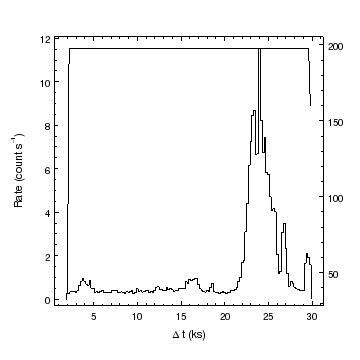### Figure 25: Adding a curve using the new Y axis

The right-hand side Y axis is used to display the new data, and has automatically increased to cover the new data range.

We change the exposure curve to use a dotted line style, so it does not distract from the histogram too much. It is no-longer necessary to call limits here to restrict the Y axis to just the data range.

```chips> set_curve(["line.style", "dot"])
```

In order to add a label to this axis we increase the rightmargin attribute of the plot from 0.1 to 0.15 and then add a label to the axis. The result is shown in Figure 26.

```chips> print(get_plot())
bottommargin = 0.15000000596
corner.style = miter
id = None
leftmargin = 0.15000000596
rightmargin = 0.10000000149
stem = None
style = closed
title.angle = 0.0
title.color = default
title.depth = 100
title.font = helvetica
title.fontstyle = normal
title.halign = 0.5
title.size = 16
title.valign = 0.5
title.xpos = 0.5
title.ypos = 15.0
topmargin = 0.10000000149

chips> set_plot(["rightmargin", 0.15])
chips> set_plot_ylabel("Exposure per bin (s)")
```

### Figure 26: Labeling the new Y axis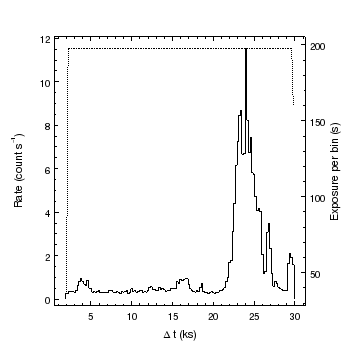### Figure 26: Labeling the new Y axis

The right-hand margin of the plot has been increased in order to make space for the axis label of "Exposure per bin (s)".

There are many attributes controlling the appearance and behavior of an axis: below we show those relevant to the axis label and slightly increase the offset between the axis and the label.

```chips> print(get_yaxis().label)
color = default
font = helvetica
fontstyle = normal
halign = 0.5
size = 14
valign = 0.0
visible = True

chips> print(get_yaxis().y)
label.angle = 90.0
label.text = Exposure per bin (s)
stem = None

chips> print(get_yaxis().offset)
parallel = 0.0
perpendicular = 40.0

chips> set_yaxis(["offset.perpendicular", 45])
```

We can switch between the Y axes - and hence coordinate systems - using the current_axis call. Below we switch back to the original Y axis and change the limits to highlight the low-count rate region. The exposure curve is unaffected by this. We then change the curve and histogram to improve the visibility of the plot, creating Figure 27.

```chips> current_axis("ay1")
chips> print(info_current())
Window [win1]
Frame [frm1]
Plot [plot1]
X Axis [ax1]
Y Axis [ay1]
Histogram [hist1]
Curve [crv1]
Coord Sys [Data]
Coord Sys ID [ds7.7.9.30]

chips> limits(Y_AXIS, 0, 2)
chips> set_histogram(["fill.style", "solid", "fill.color", "skyblue"])
chips> set_curve(["line.color", "red", "line.thickness", 3])
chips> set_histogram(["depth", 50])
```

### Figure 27: Switching back to the original Y axis### Figure 27: Switching back to the original Y axis

The y axis has been zoomed in from the original range of close to 0 to 15 to highlight the low count-rate region. Note that the exposure axis has not changed from that shown in Figure 26.

Since both the curve and the histogram share the same X axis, the following command zooms both data sets and changes the axis labels to match the data they display. The result is Figure 28.

```chips> limits(X_AXIS, 3, 20)
chips> save_state("axis2-xzoom.state")
chips> print(info_coordinate())
Window [win1]
Frame [frm1]
Plot [plot1]
Coord Sys ID [ds7.7.9.30]
X Axis [ax1]
Y Axis [ay1]
Coord Sys ID [ds7.7.9.31]
X Axis [ax1]
Y Axis [ay2]

chips> set_yaxis(["label.color", "skyblue"])
chips> set_yaxis("ay2", ["label.color", "red"])
```

### Figure 28: Changing the X-axis limits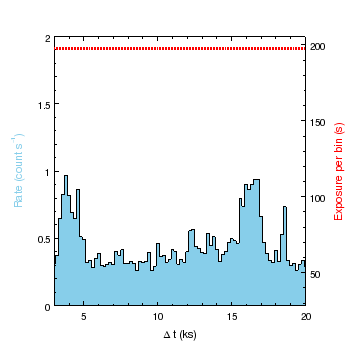### Figure 28: Changing the X-axis limits

As both coordinate systems - ds7.7.9.30 which shows the count rate data and ds7.7.9.31 which shows the exposure data - use the same X axis (ax1), changing the limits of this axis changes both the curve and histogram.

## Support for irregularly-spaced bins

The low and high edges of consecutive histogram bins do not have to match, nor do the bins have to have the same widths, as shown in Figure 29:

```chips> clear()
chips> xlo = [1, 3, 5, 9, 11, 14]
chips> xhi = [2, 5, 9, 10, 14, 15]
chips> y = [1e4, 6e4, -2e4, 1.2e5, 7e3, 9e3]
chips> add_histogram(xlo, xhi, y)
```

### Figure 29: Support for irregularly-binned data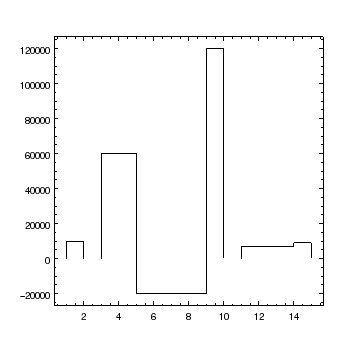### Figure 29: Support for irregularly-binned data

The histogram bins do not need to be regularly spaced, and there can be gaps between bins. The Y values can also be zero or negative.

The normal attributes of a histogram work as expected with this data, as shown in Figure 30, where a number of attributes have been changed. We also change the format used for the tick labels on the Y axis.

```chips> hi = ChipsHistogram()
chips> hi.fill.style = "solid"
chips> hi.fill.color = "green"
chips> hi.line.color = "red"
chips> hi.symbol.style = "diamond"
chips> hi.symbol.fill = True
chips> hi.symbol.color = "blue"
chips> hi.symbol.size = 7
chips> hi.dropline = 1
chips> set_histogram(hi)
chips> set_yaxis(["tickformat", "%.5Z"])
```

### Figure 30: Changing many histogram attributes### Figure 30: Changing many histogram attributes

The bins are filled down (or up) to the y=0 line, and marked by a symbol at the mid point of each bin. We make use of the ticklabel format support to use exponential notation for large values (an absolute value of 105 or greater).

## Summary

• Histograms can be created from data consisting of either:

1. xmid, y
2. xlow, xhigh, y

and can include errors on the y values (as symmetric or asymmetric values).

• Histograms can be drawn using lines, symbols or both. The lines are drawn at the y value across the bin width whereas the symbols are drawn at the mid-point of each bin.

• Histograms can be drawn using a solid fill, whose opacity can be varied between 0 and 1 inclusively. The fill color extends down to, or up to, the y=0 value as shown in Figure 30). The fill need not be a solid color, since a range of fill patterns is supported (e.g. Figure 8).

All output formats except for PS and EPS support opacity and alpha settings (e.g. compare the different formats in Figure 6). There are still issues in CIAO 4.8 with the PS and PDF output, where filled areas may show faint lines rather than appear as a solid color when displayed on screen (this should not be visible on print out).

• Histograms and curves can be drawn in the same plot, as shown in Figure 3 and Figure 26.

• Some commands, such as set_plot_xlabel and set_plot_ylabel, take an optional first argument which defines the plot to act on. This then results in the currency being changed to the plot. This means that

```chips> set_plot_ylabel("plot2", "v_{circ} (m s^{-1})")
```

is equivalent to

```chips> current_plot("plot2")
chips> set_plot_ylabel("v_{circ} (m s^{-1})")
```
• Axes can be adjusted to improve the legibility of the ticklabels. There are five different settings that can be used for the majortick.mode attribute of an axis: limits, count, interval, nice, and arbitrary. These modes determine how the major tick positions are computed and what data range to display. Examples of the count mode and interval mode are shown in Figure 11 and Figure 10 respectively.

• The pick and get_pick commands can be used to inspect a plot. The former prints the coordinate of the selected points to the screen whereas the latter returns the values in arrays.

The ChIPS GUI provides interactive capabilities for zooming and panning.

• Plots need not be arranged in a grid, as shown in Figure 2, but can be manually added to any position within a frame, using add_plot. They can even be placed "within" another plot, as shown in Figure 23.

• Data which share one common axis can be drawn on the same plot by adding an extra axis. An example of this is shown in Figure 25, where count rate data and exposure time data are both plotted on the Y axis against the same time axis (in this case given as the offset since the start of the observation).

• For objects like axes, curves and histograms, which contain attributes of the form "a.b", the "*.b" format can be used to change those attributes like color and thickness which appear multiple times. So

```chips> set_histogram(["*.color", "red"])
```

will change the line.color, symbol.color and err.color attributes of the current histogram.

Note that the set_cascading_property command can be used to change all child objects, so

```chips> set_cascading_property(chips_plot, "color", "red")
```

changes all color attributes of the current plot, and all of its children such as any histograms, axes or curves, to red.

## History

 09 Jul 2008 New for CIAO 4.0 15 Dec 2008 CIAO 4.1 - use copy_colvals rather than get_colvals; histograms can now be filled by a range of patterns; the pick_limits command has been added 15 Dec 2009 Updated for CIAO 4.2: the support for filled and opaque regions and histograms has improved for PNG and JPEG output (see Figure 5 and Figure 6), converted to use the *.color attribute rather than the set_cascading_property command when creating Figure 23, it is no longer necessary to call limits(Y_AXIS,AUTO,AUTO) after adding the second Y axis when creating Figure 25. 15 Dec 2010 Updated for CIAO 4.3: the lightcurve (lc.fits) has been re-generated using dmextract so that we can use the new DT virtual column in the plots. The plots have also been changed to use the COUNT_RATE rather than COUNTS column. 15 Dec 2011 Updated for CIAO 4.4: noted that opacity support has been added to PDF output (e.g. Figure 6); added notes about the new ChIPS GUI. 13 Dec 2012 Updated for CIAO 4.5 04 Dec 2013 Updated for CIAO 4.6: noted the change in behavior with axis labels (Figure 26) and the new x.label and y.label attributes for axes. 10 Dec 2014 Updated for CIAO 4.7. 15 Dec 2015 Updated for CIAO 4.8.